# 图像的灰度增强、去噪、图像增强

### futurehello博客

http://blog.csdn.net/xixi_0921/article/details/50533487  整理笔记

S=T(r);

(1)改善图像的质量，使图像能够显示更多的细节，提高图像的对比度（对比度拉伸）
(2)有选择的突出图像感兴趣的特征或者抑制图像中不需要的特征
(3)可以有效的改变图像的直方图分布，使像素的分布更为均匀

# 二、灰度变换增强的方法及实现

• 线性函数 （图像反转）
• 对数函数:对数和反对数变换
• 幂律函数：n次幂和n次开方变换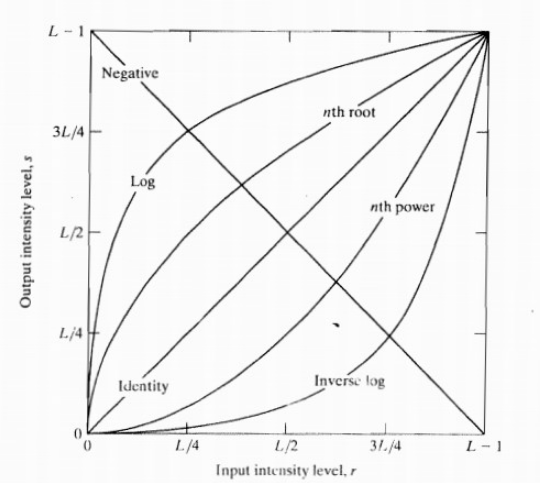### 线性变换

• ,增加图像的对比度
• ,减小图像的对比度
• ，图像整体的灰度值上移或者下移，也就是图像整体变亮或者变暗，不会改变图像的对比度。
• ，图像的亮区域变暗，暗区域变亮
• ,恒定变换，不变
• 图像反转。

a=imread('circlesBrightDark.png');          %读取原始图像
subplot(1,2,1),
imshow(a);                 %显示原始图像
title('原始图像');
%显示函数的曲线图
b=255-a;
subplot(1,2,2),
imshow(b)
title('反转之后的图像');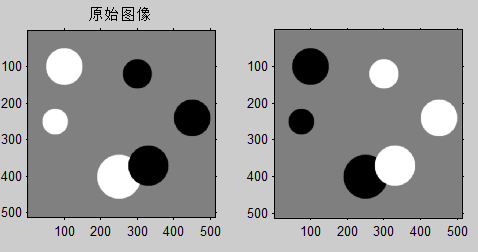I =imread('lena.jpg');
[d1,d2,d3] =size(I);
if(d3 > 1)
I =rgb2gray(I);%如果是灰度图就不用先变换
end
Im = double(I);
A=0.5; B=0;
darker=Im*A+B;
A=1;B=0;
mover=Im*A+B;
A=1.5;B=0;
lighter=Im*A+B;
A=-1;B=255;
reverser=Im*A+B;
J1=uint8(darker);
J2=uint8(mover);
J3=uint8(lighter);
J4=uint8(reverser);
subplot(2,3,1),imshow(I);title('原图');
subplot(2,3,2),imshow(J1);title('a=0.5 减小对比度');
subplot(2,3,5),imshow(J2);title('a=1灰度值上移');
subplot(2,3,3),imshow(J3);title('a=1.5增大对比度');
subplot(2,3,6),imshow(J4);title('a=-1反相');

### 分段线性变换（matlab的灰度变换函数）

I=imread('glass.png');      	%读取图像
subplot(2,2,1);
imshow(I);
title('原图像');
subplot(2,2,2);
imhist(I);
title('原图像直方图');
subplot(2,2,3);
imshow(J);
title('调整灰度后的图像');
subplot(2,2,4);
imhist(J);
title('调整灰度后的直方图');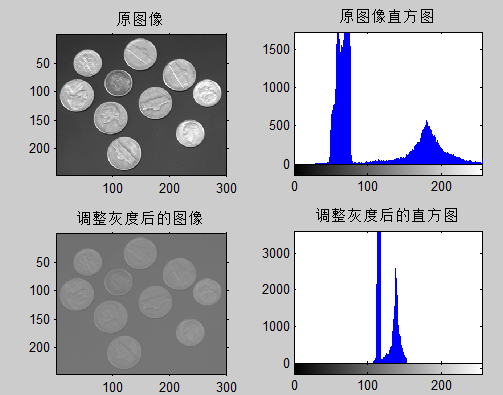# gamma校正背景

在电视和图形监视器中，显像管发生的电子束及其生成的图像亮度并不是随显像管的输入电压线性变化，电子流与输入电压相比是按照指数曲线变化的，输入电压的指数要大于电子束的指数。这说明暗区的信号要比实际情况更暗，而亮区要比实际情况更高。所以，要重现摄像机拍摄的画面，电视和监视器必须进行伽玛补偿。这种伽玛校正也可以由摄像机完成。我们对整个电视系统进行伽玛补偿的目的，是使摄像机根据入射光亮度与显像管的亮度对称而产生的输出信号，所以应对图像信号引入一个相反的非线性失真，即与电视系统的伽玛曲线对应的摄像机伽玛曲线，它的值应为1/γ，我们称为摄像机的伽玛值。电视系统的伽玛值约为2.2，所以电视系统的摄像机非线性补偿伽玛值为0.45。彩色显像管的伽玛值为2.8，它的图像信号校正指数应为1/2.8=0.35，但由于显像管内外杂散光的影响，重现图像的对比度和饱和度均有所降低，所以彩色摄像机的伽玛值仍多采用0.45。在实际应用中，我们可以根据实际情况在一定范围内调整伽玛值，以获得最佳效果。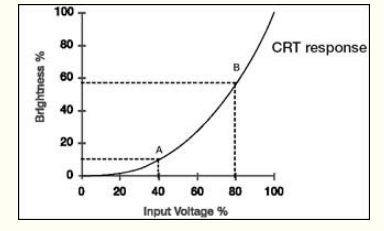## gamma校正定义

(Gamma Correction，伽玛校正）：所谓伽玛校正就是对图像的伽玛曲线进行编辑，以对图像进行非线性色调编辑的方法，检出图像信号中的深色部分和浅色部分，并使两者比例增大，从而提高图像对比度效果。计算机绘图领域惯以此屏幕输出电压与对应亮度的转换关系曲线，称为伽玛曲线（Gamma Curve）。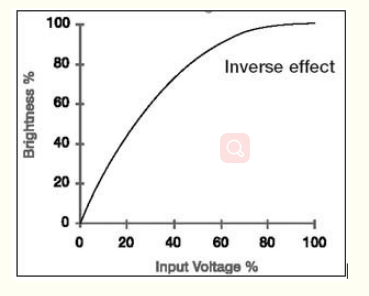## gamma校正原理

假设图像中有一个像素，值是 200 ，那么对这个像素进行校正必须执行如下步骤：

1. 归一化 ：将像素值转换为  0 ～ 1  之间的实数。 算法如下 : ( i + 0. 5)/256  这里包含 1 个除法和 1 个加法操作。对于像素  A  而言  , 其对应的归一化值为  0. 783203 。

2. 预补偿 ：根据公式  , 求出像素归一化后的 数据以  1 /gamma  为指数的对应值。这一步包含一个 求指数运算。若  gamma  值为  2. 2 ,  则  1 /gamma  为  0. 454545 , 对归一化后的  A  值进行预补偿的结果就 是  0. 783203 ^0. 454545 = 0. 894872 。

3. 反归一化 ：将经过预补偿的实数值反变换为  0  ～  255  之间的整数值。具体算法为 : f*256 - 0. 5  此步骤包含一个乘法和一个减法运算。续前 例  , 将  A  的预补偿结果  0. 894872  代入上式  , 得到  A  预补偿后对应的像素值为  228 , 这个  228  就是最后送 入显示器的数据。

如上所述如果直接按公式编程的话，假设图像的分辨率为 800*600 ，对它进行 gamma 校正，需要执行 48 万个浮点数乘法、除法和指数运算。效率太低，根本达不到实时的效果。

针对上述情况，提出了一种快速算法，如果能够确知图像的像素取值范围  , 例如  , 0 ～ 255 之间的整数  , 则图像中任何一个像素值只能 是  0  到  255  这  256  个整数中的某一个 ; 在  gamma 值 已知的情况下  ,0 ～ 255  之间的任一整数  , 经过“归一 化、预补偿、反归一化”操作后 , 所对应的结果是唯一的  , 并且也落在  0 ～ 255  这个范围内。

如前例  , 已知  gamma  值为  2. 2 , 像素  A  的原始值是  200 , 就可求得 经  gamma  校正后  A  对应的预补偿值为  228 。基于上述原理  , 我们只需为  0 ～ 255  之间的每个整数执行一次预补偿操作  , 将其对应的预补偿值存入一个预先建立的  gamma  校正查找表 (LUT:Look Up Table) , 就可以使用该表对任何像素值在  0 ～ 255  之 间的图像进行  gamma  校正。

for i=0:255;
f=power((i+0.5)/256,1/2.2);
LUT(i+1)=uint8(f*256-0.5);
end
img0=rgb2ycbcr(img);
R=img(:,:,1);
G=img(:,:,2);
B=img(:,:,3);
Y=img0(:,:,1);
Yu=img0(:,:,1);
[x y]=size(Y);
for row=1:x
for width=1:y
for i=0:255
if (Y(row,width)==i)
Y(row,width)=LUT(i+1);
break;
end
end
end
end
img0(:,:,1)=Y;
img1=ycbcr2rgb(img0);
R1=img1(:,:,1);
G1=img1(:,:,2);
B1=img1(:,:,3);
subplot(1,2,1);
imshow(img);         	%显示图像
title('原图');
subplot(1,2,2);
imshow(img1);
title('Gamma矫正后的图像')

3对数函数灰度变换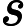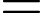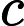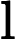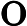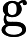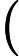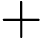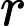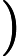I=imread('office_4.jpg');
I1=rgb2gray(I);
subplot(1,2,1),imshow(I1);
title('灰度图像');
axis([50,250,50,200]);
grid on;          	%显示网格线
axis on;       	%显示坐标系
J=double(I1);
J=40*(log(J+1));
H=uint8(J);
subplot(1,2,2),
imshow(H);
title('对数变换图像');
axis([50,250,50,200]);
grid on;
axis on;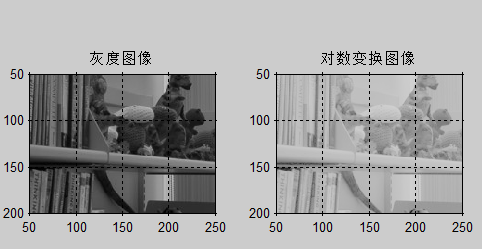===============================

Im=imread('coins.png');
f =mat2gray(Im,[0 255]);
v = 5;
g_1 = log2(1 +v*f)/log2(v+1);
v = 10;
g_2 = log2(1 +v*f)/log2(v+1);
v = 200;
g_3 = log2(1 +v*f)/log2(v+1);
subplot(2,2,1);
imshow(f,[0 1]);
xlabel('a).原图');
subplot(2,2,2);
imshow(g_1,[0 1]);
xlabel('b).\fontname{TimesNew Roman}\itv\rm=5');
subplot(2,2,3);
imshow(g_2,[0 1]);
xlabel('c).\fontname{TimesNew Roman}\itv\rm=10');
subplot(2,2,4);
imshow(g_3,[0 1]);
xlabel('d).\fontname{TimesNew Roman}\itv\rm=200');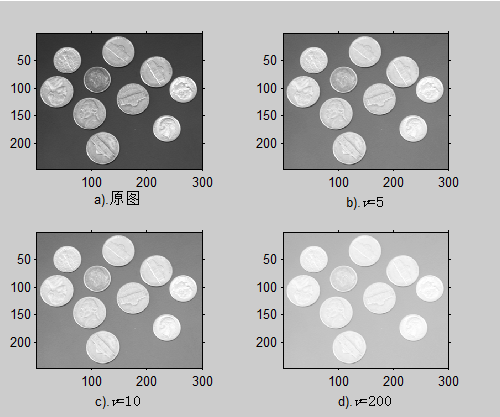4.反对数变换

Im =imread('coins.png');
f =mat2gray(Im,[0 255]);
v = 2;
g_1 =((f.^(v+1)))/v;
disp(g_1);
v = 5;
g_2 =((f.^(v+1)))/v;
disp(g_2);
v = 20;
g_3 =((f.^(v+1)))/v;
subplot(2,2,1);
imshow(f,[0 1]);
xlabel('a).原图');
subplot(2,2,2);
imshow(g_1,[0 1]);
xlabel('b).\fontname{TimesNew Roman}\itv\rm=2');
subplot(2,2,3);
imshow(g_2,[0 1]);
xlabel('c).\fontname{TimesNew Roman}\itv\rm=5');
subplot(2,2,4);
imshow(g_3,[0 1]);
xlabel('d).\fontname{TimesNew Roman}\itv\rm=20');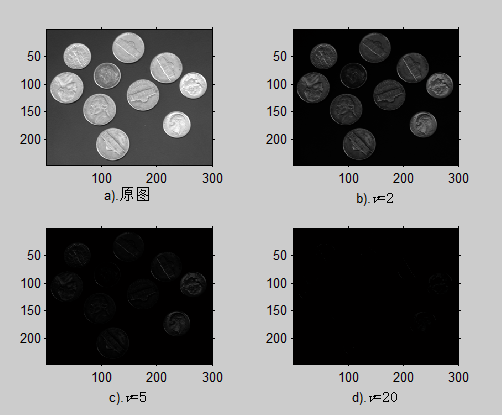5.直方图均衡化

http://blog.csdn.net/superjunenaruto/article/details/52431941  写的非常好的一个博客，通俗易懂。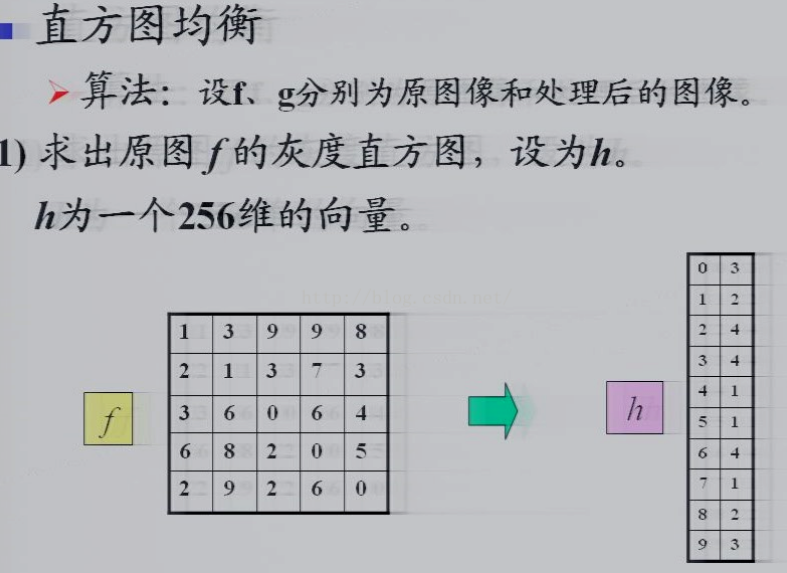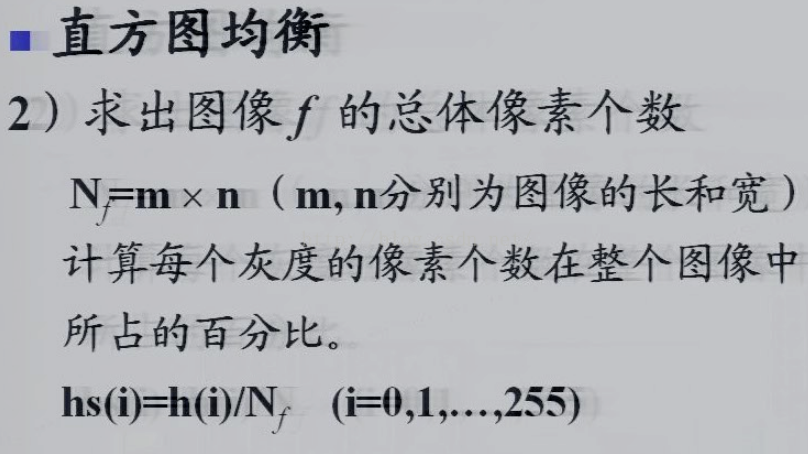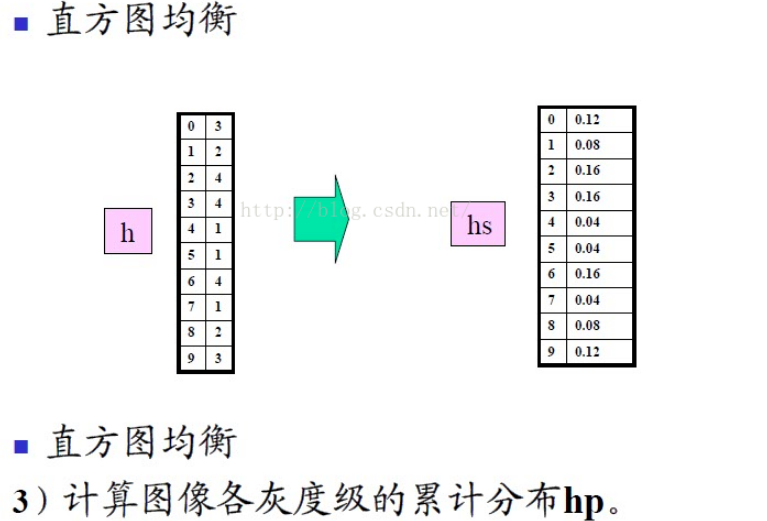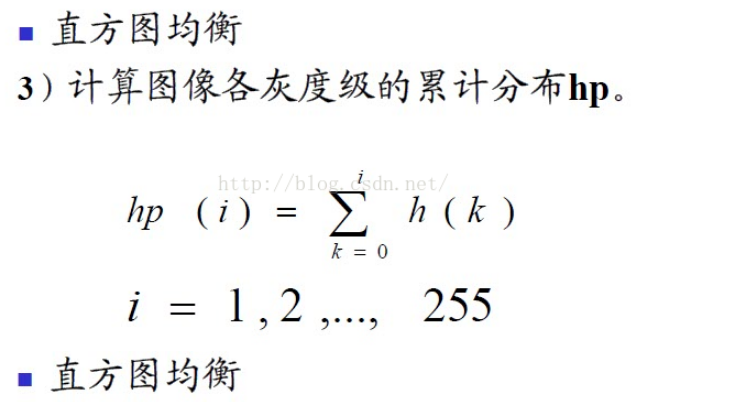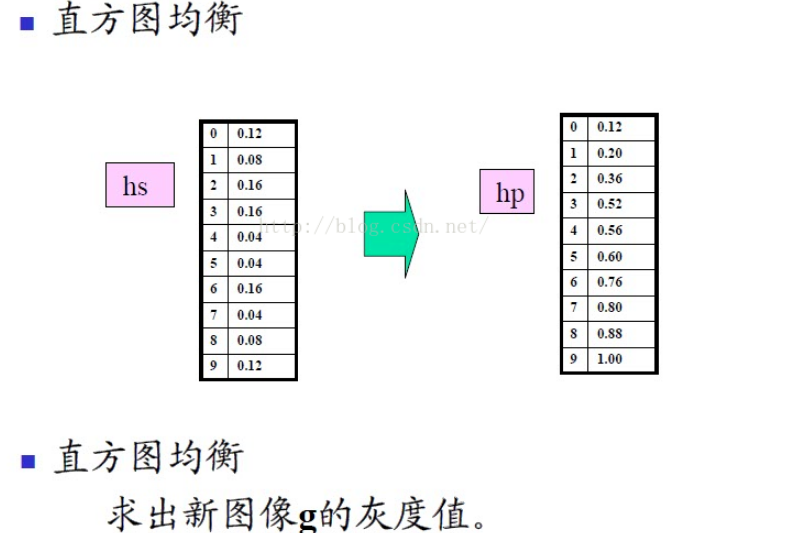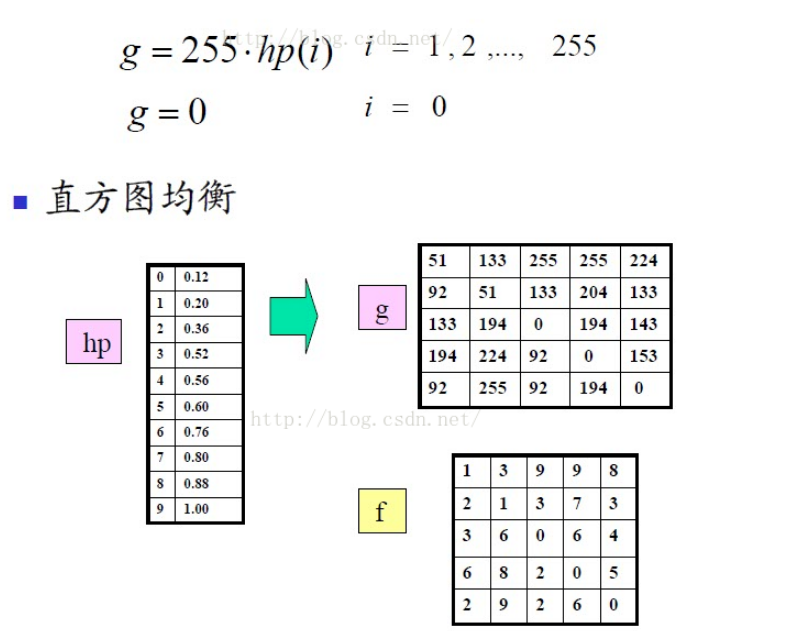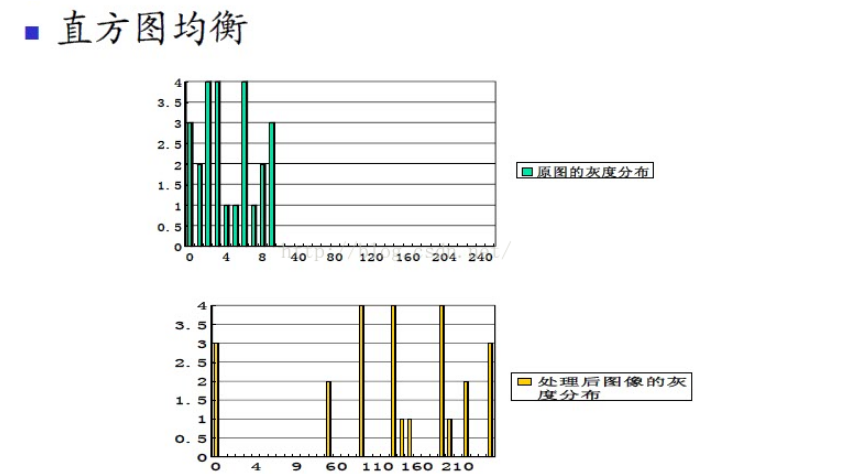I =imread('coins.png');
[d1,d2,d3] =size(I);
if(d3 > 1)
I = rgb2gray(I);%如果是灰度图就不用先变换
end
I = double(I) /255;
Im = uint8(255 *I * 0.5 + 0.5);
subplot(2,2,1);imshow(Im);title('原图');
gp=zeros(1,256);%计算各灰度出现的概率
for i=1:256
gp(i)=length(find(Im==(i-1)))/(d1*d2);
end
subplot(2,2,2);
bar(0:255,gp);
set(gca,'XLim',[0 255]);
title('原图灰度直方图');
newGp=zeros(1,256);%计算新的各灰度出现的概率
S1=zeros(1,256);
S2=zeros(1,256);
tmp=0;
for i=1:256
tmp=tmp+gp(i);
S1(i)=tmp;
S2(i)=round(S1(i)*256);
end
for i=1:256
newGp(i)=sum(gp(find(S2==i)));
end
subplot(2,2,4);
bar(0:255,newGp);
set(gca,'XLim',[0 255]);
title('均衡化后直方图');
newGrayPic=Im; %填充各像素点新的灰度值
for i=1:256
newGrayPic(find(Im==(i-1)))=S2(i);
end
subplot(2,2,3);imshow(newGrayPic);title('均衡化后');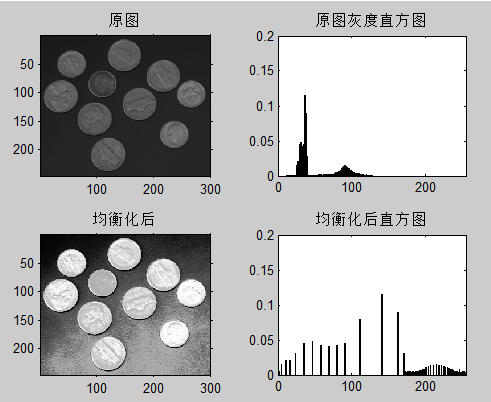(1)输出图像的实际灰度变化范围很难达到图像格式所允许的最大灰度变化范围；

(2)输出图像的灰度分布直方图虽然接近均匀分布, 但其值与理想值1/n仍有可能存在较大的差异, 并非是最佳值；

(3)输出图像的灰度级有可能被过多地合并。由于灰度的吞噬也易造成图像信息的丢失。

10-28161401-174万+
09-15322
04-202556
09-164万+
12-151万+
08-23
07-114万+
08-131万+
03-04